### 树脂复合钩盖板

#### 作者：江苏常州巨龙建筑材料厂 来源：本站 发布日期：2021-07-06 14:27:25 点击次数：2607

350*250*30=12

300*400*30=15

300*450*40=22

500*200*30=20

500*250*30=22

500*300*30=25

500*300*40=28

500*400*30=20

500*400*40=32

500*500*40=35

500*500*50=60

600*400*40=42

600*400*50=60

380*680*40=45

380*680*50=60

750*450*40=50

750*450*50=90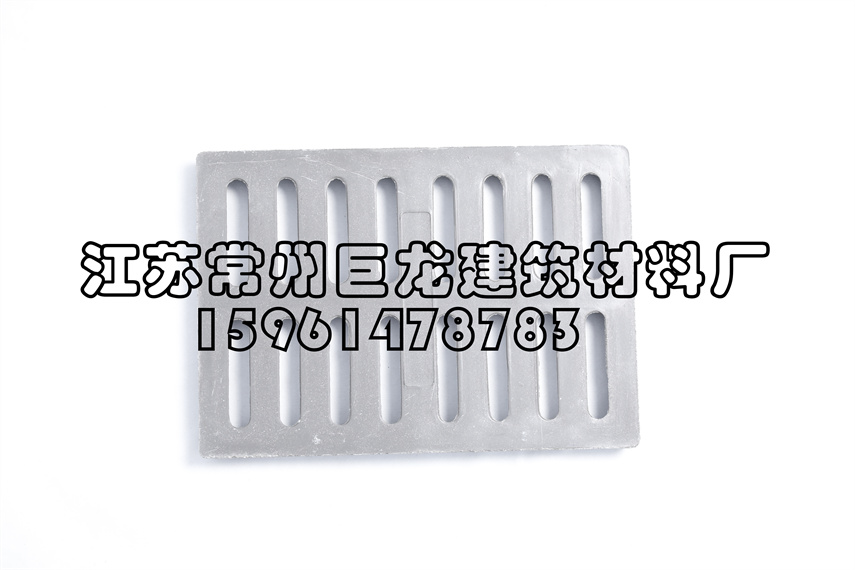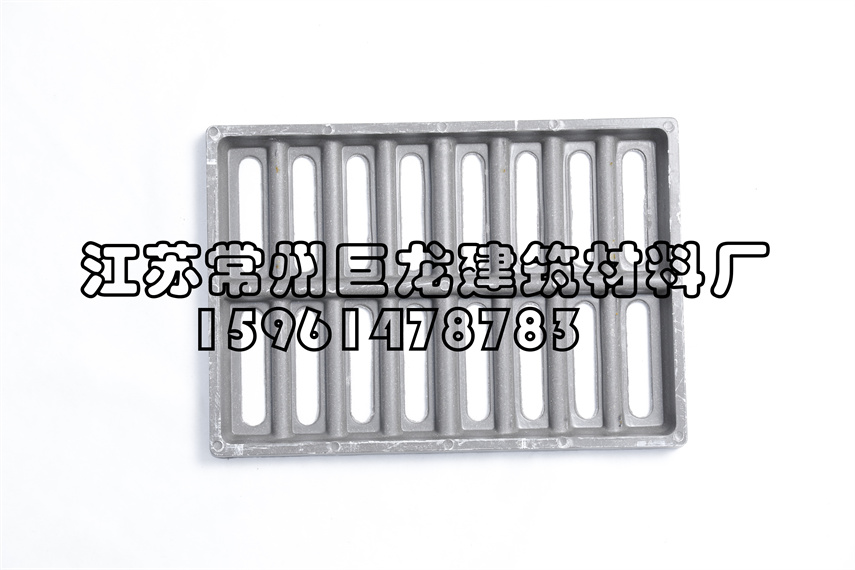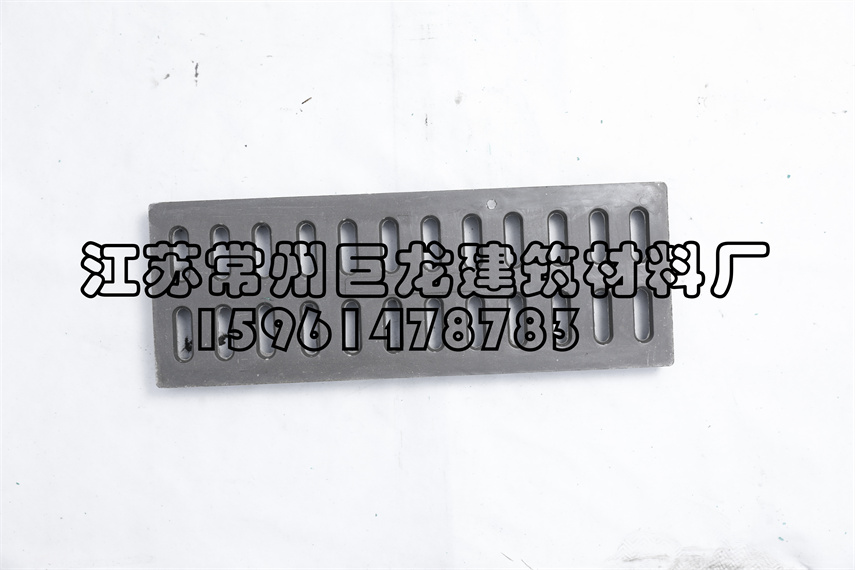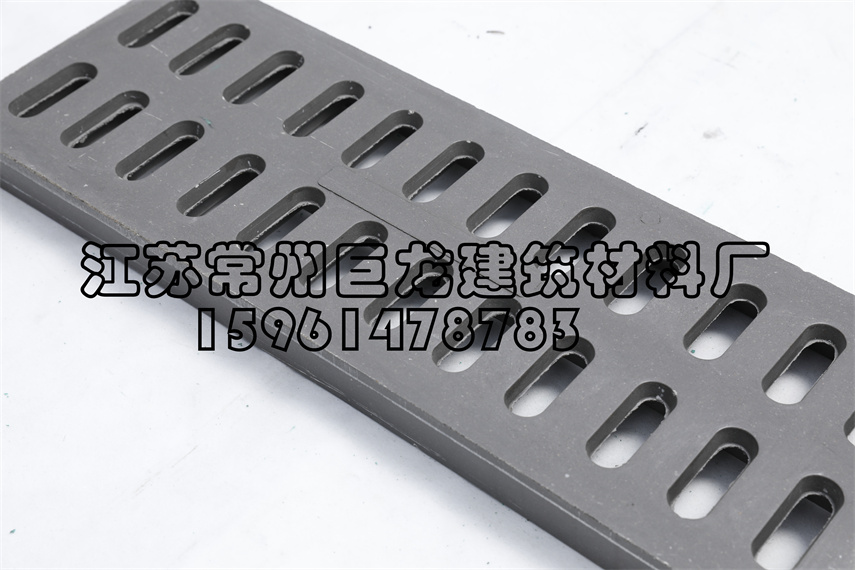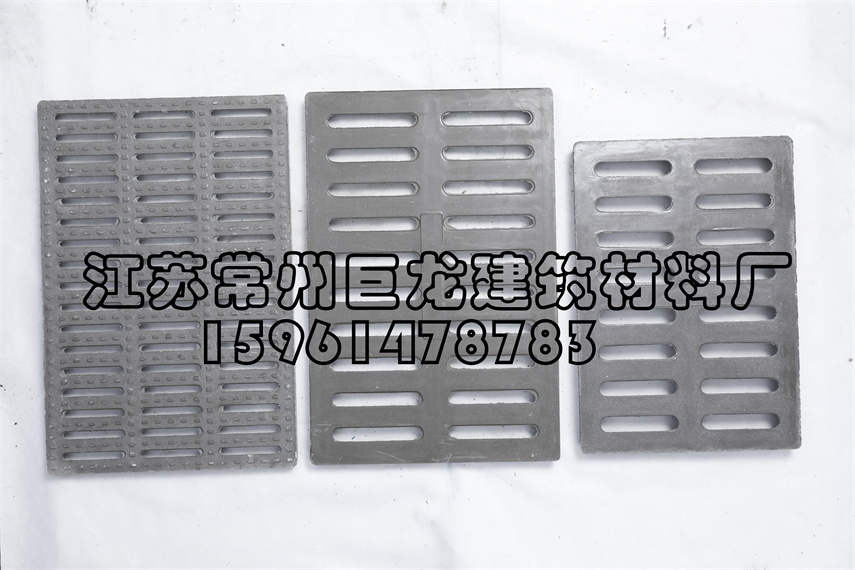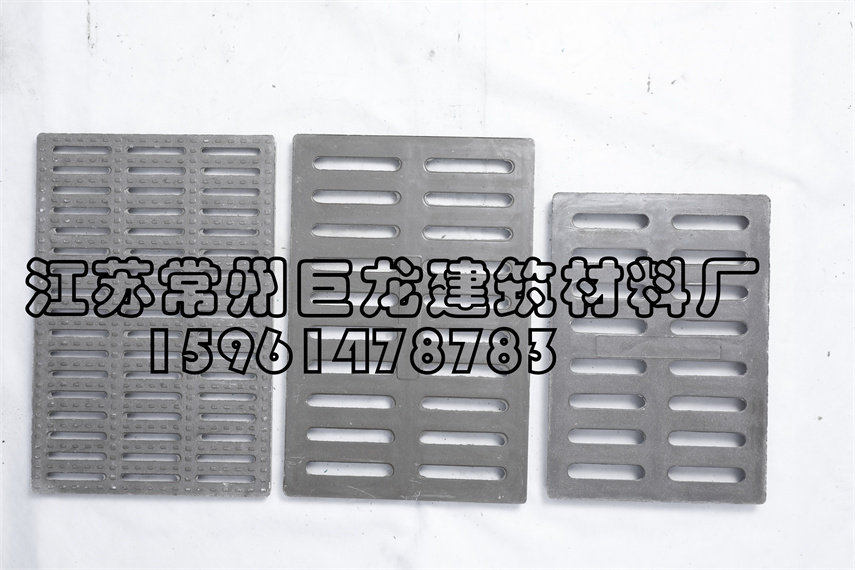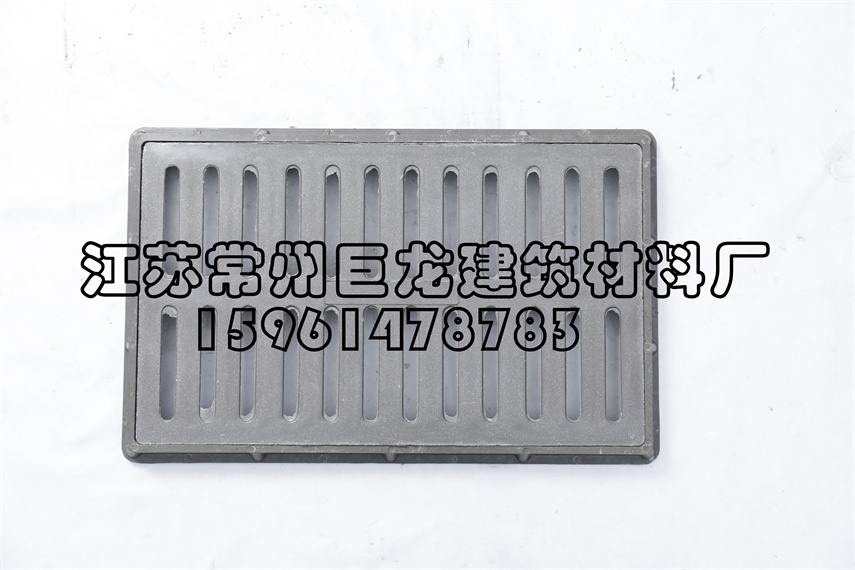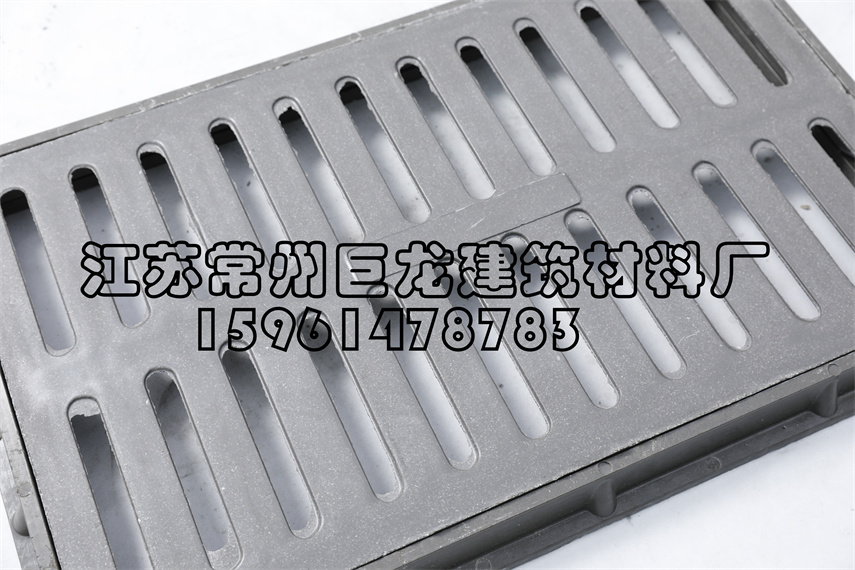• 相关标签
 公司名称： 联系人： 联系电话： 联系邮箱： 联系地址： 备注：
##### 联系我们

 姓名： 电话： 留言：

• 15961478783
•• 点击扫描下载